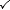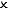# First lawThermodynamics is the study of the movement of heat and its relationship with work. Throughout this topic of thermodynamics, we need to agree on a few key terms.

Key Concepts

Thermal energy is an energy store in a body of matter. Zoom in far enough, and thermal energy becomes the sum of the kinetic energy of the individual particles.

Heat is an energy pathway, a mechanism by which energy can be transferred from one body to another.

Work is the product of the force exerted by a body and the distance travelled parallel to the force.We will refer to three types of system:

System type Heat can pass in and out? Work can be done? Matter exchange with surroundings? Example
OpenKettle with spout
ClosedGas syringe
IsolatedPerfect vacuum flask (or the universe!)

First law of thermodynamics

If heat enters a gas container, the gas can respond in one of three ways.

#### Temperature rise

The gas may increase in temperature, with a consequential increase in the pressure acting on the walls of the container. This is an increase in internal energy. Internal energy is defined as the sum of the kinetic and potential energies of the molecules in the container:

$$U=\sum{E_\textrm{K}}+\sum{E_\textrm{P}}$$

Modelling the gas as ideal, with no intermolecular forces and hence no potential energy: $$U=\sum{E_\textrm{K}}$$

We can recall from gases that the mean kinetic energy of the gas molecules is proportional to temperature: $$\bar{E_\textrm{K}}={3\over2} kT$$

The change in internal energy is equal to the total change in kinetic energy of the molecules.

$$\Delta U=N\Delta \bar{E_\textrm{K}}={3\over 2}Nk\Delta T={3\over 2}nR\Delta T$$

• $$\Delta U$$ is change in internal energy (J)
• $$N$$ is the number of molecules
• $$\bar{E_\textrm{K}}$$ is the average kinetic energy of the molecules (J)
• $$k$$ is the Boltzmann constant (=1.38 x 10-23 JK-1)
• $$\Delta T$$ is change in temperature (K)
• $$n$$ is number of moles (mol)
• $$R$$ is the ideal gas constant (=8.31 Jmol−1K−1)

#### Expansion

An expansion of the gas container into the surroundings may occur, to maintain a constant pressure. This is work done. Work done is the product of the force exerted by a body and the distance travelled parallel to the force:

$$W=Fs\cos \theta$$

The force emerges from the combined pressure of the collisions by gas molecules across the surface area of the walls of the container: $$F=pA$$

Taking the surface area of the container as constant (e.g. piston), the displacement of the container walls will be perpendicular to the plane of the walls. This results in a change in volume: $$\Delta V=As$$

With the expansion at constant pressure:

$$W=p\Delta V$$

• $$W$$ is work done by the gas on the surroundings (J)
• $$p$$ is the pressure of the gas (Pa)
• $$\Delta V$$ is the change in volume of the container (m3)

Essentials

The first law of thermodynamics combines changes in temperature and work done. It is a restatement of conservation of energy:

The amount of heat that enters a closed gas container is equal to the sum of the increase in internal energy and the work done by the gas on the surroundings.

$$Q=\Delta U+W$$

• $$Q$$ is heat (J), with $$Q> 0$$ for heat entering the gas container
• $$\Delta U$$ is change in internal energy (J), with $$\Delta U >0$$ for an increase in the internal energy of the gas
• $$W$$ is work done (J), with $$W>0$$ for work done on the surroundings by the gas

There is a logical flow to the equation when expressed with respect to $$Q$$.

Summary

Have a go at making a thermodynamic change using this Geogebra simulation.

Test Yourself

Use flashcards to practise your recall.

Use quizzes to practise application of theory.

Exam-style Questions

Online tutorials to help you solve original problems